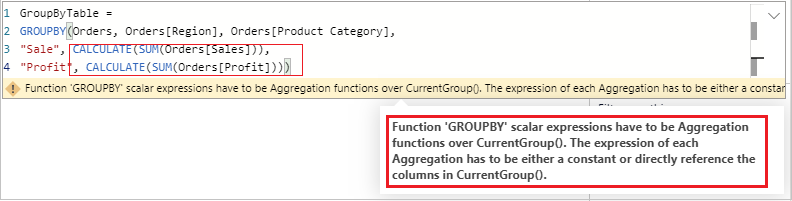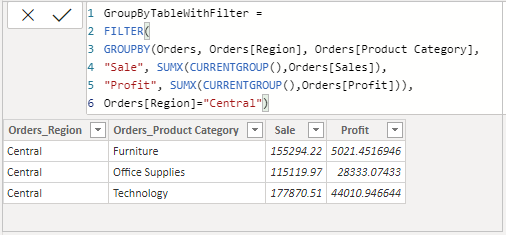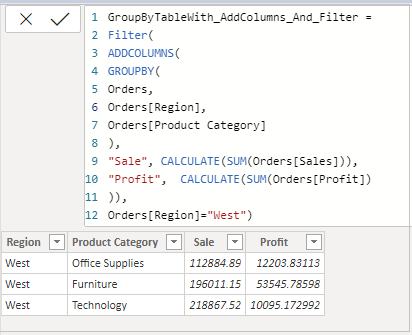Home » DAX » DAX – GROUPBY Function

# DAX – GROUPBY FunctionReturns a table with a set of selected columns. It comes under Table Manipulation DAX Functions category.

GROUP BY permits DAX CURRENTGROUP function to be used inside aggregation functions in the extension columns that it adds.

It attempts to reuse the data that has been grouped making it highly performant.

DAX GROUPBY function is similar to DAX SUMMARIZE function. However, GROUPBY does not do an implicit CALCULATE for any extension columns that it adds.

#### Syntax:

`GROUPBY (<table>, [<groupBy_columnName1>], [<name>, <expression>]… )`

#### Description:

 S no. Parameter Description 1 table Any DAX expression that returns a table of data. 2 groupBy_columnName (Optional) A column to group by. 3 name A column name to be added, enclosed in double quotes. 4 expression Any DAX expression that returns a single scalar value, where the expression is to be evaluated for each set of GroupBy values.

#### Note:

1. The expression used in GroupBy may include any of the “X” aggregation functions, such as SUMX, AVERAGEX, MINX, MAXX, etc.
2. DAX CALCULATE function and calculated fields are not allowed in the expression.
3. groupBy_columnName must be either in table or in a related table.
4. Each name must be enclosed in double quotation marks.
5. This function is not supported for use in DirectQuery mode when used in calculated columns or row-level security (RLS) rules.
6. CURRENTGROUP function can only be used in an expression that defines a column within the GROUPBY function.

So, let’s begin with an example. You can download the sample dataset from the link provided below.

Create new table, Go to Modeling tab and click to Table

### GROUPBY does not support Calculate:-

```GroupByTable =
GROUPBY(Orders, Orders[Region], Orders[Product Category],
"Sale", CALCULATE(SUM(Orders[Sales])),
"Profit", CALCULATE(SUM(Orders[Profit])))```GroupByDAX

#### It supports only “X” aggregations function with CURRENTGROUP DAX:-

```GroupByTable =
GROUPBY(Orders, Orders[Region], Orders[Product Category],
"Sale", SUMX(CURRENTGROUP(),Orders[Sales]),
"Profit", SUMX(CURRENTGROUP(),Orders[Profit]))```GroupByDaxCurrentGroup

#### Working of Nested GROUPBY:-

It will return MAX Product Category Sales region wise.

```GroupByTable =
GROUPBY(
GROUPBY(Orders, Orders[Region], Orders[Product Category],
"TotalSale", SUMX(CURRENTGROUP(),Orders[Sales])
),
Orders[Region],
"MAX Sales", MAXX(CURRENTGROUP(),[TotalSale]))```NestedGroupBY

#### GROUPBY with Filter:-

```GroupByTable =
FILTER(
GROUPBY(Orders, Orders[Region], Orders[Product Category],
"Sale", SUMX(CURRENTGROUP(),Orders[Sales]),
"Profit", SUMX(CURRENTGROUP(),Orders[Profit]))),
Orders[Region]="Central")```GroupByDAXWithFilter

#### GROUPBY with ADDCOLUMNS, support CALCULATE:-

```GroupByTableWithAddColumns =
GROUPBY(Orders,
Orders[Region],
Orders[Product Category]),
"Sale", CALCULATE(SUM(Orders[Sales])),
"Profit", CALCULATE(SUM(Orders[Profit]))
)```

```GroupByTableWith_AddColumns_And_Filter =

FILTER(
GROUPBY(Orders,
Orders[Region],
Orders[Product Category]
),
"Sale", CALCULATE(SUM(Orders[Sales])),
"Profit", CALCULATE(SUM(Orders[Profit])
)),
Orders[Region]="West")```